Home > CCA2 > Chapter 10 > Lesson 10.3.1 > Problem10-162

10-162.
1. Solve each equation below. Homework Help ✎

1. 2(x−1) = 64

2. 93 = 27(2x − 1)

3. x6 = 29

4. 6x = 29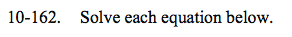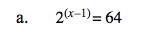2? = 64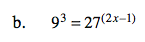Change the left side of the equation to:

93 = (32)3 = 36

Change the right side of the equation to a power of 3, also.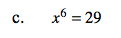How can you 'undo' a 6th power?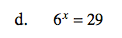Since the x is in the exponent, you will need to take the log of both sides.